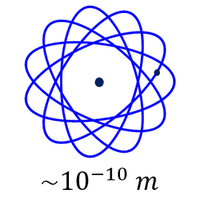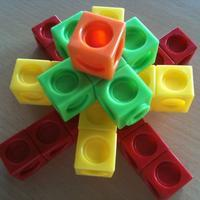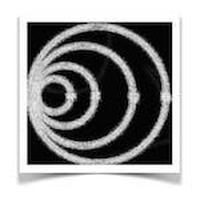# Number and Algebra

##Numbers and the world around us!

In this section of the syllabus, we have topics about the number systems and notation that we use to describe the world around us, the notions of accuracy, estimation and percentage error and sequences which cross over with the Functions and Modelling section. How much of what know about the size of is an estimation? How accurate does an estimation need to be before it has value? How and why do we work with really big and really small numbers? How do things grow or shrink over time if they follow patterns of growing by the same amount of the same multiple each time? For example how can we estimate the spread of infectious diseases?

## What is in this section?

#### 1.1 Standard Index Form

This is a small sup-topic to introduce or recap numbers in standard form. We will learn how to convert between numbers in standard form and base form, alongside learning how to operate with this succinct and helpful way to express numbers.

#### 1.2 Arithmetic Sequences and Series

These are sequences where the difference from one term to the next remains constant, like the one in the title! Arithmetic sequences crop up in all sorts of places and this unit looks at understanding how to generalise, sum and solve problems...

#### 1.3 Geometric Sequences

These are sequences where you go from term to term by multiplying by a common ratio. The one in the title has a common ratio of 3 because each term is 3 times bigger than the previous one. Geometric sequences grow exponentially and should be...

#### 1.6 Accuracy and Estimation

Accuracy and Estimation  When numbers are reported in the news, or as statistical results, they are either displayed exactly, or they are rounded to a certain degree of accuracy. Understanding how and when to do this can give us a deeper insight...

#### Prior Knowledge - Sets of Numbers

This sub topic is about the 4 key sets of numbers that define our number system. Natural numbers, Integers, Rational numbers and Real numbers. This section starts by taking you through the concept of number sets, starting with sets that we...

## Key Questions

The following are list of key questions that you might be asking about these topics with links to the places where you can get the answers!

## How do you round to 3 significant figures?

In this IB course, we are most often expected to round our answers to three significant figures. Learn how:  1.6 Accuracy and Estimation

## What is percentage error?

There is a big difference between percentage error and absolute error - it can tell us a lot more about the world! Find out how to calculate it. 1.6 Accuracy and Estimation

## How do you write in standard form?

Standard form is used to express very large or very small numbers, and it is important that we can work out what it means and calculate with it. Practice these skills here:  1.1 Standard Index Form

## What is an arithmetic sequence?

An arithmetic sequence is one which changes in a linear fashion. It exists in plenty of real life situations and we can use our understanding of it to solve many problems!  Find out more:  1.2 Arithmetic Sequences

## How do you know if a sequence is geometric?

A sequence is geometric if it has a common ratio between terms. They are find frequently when looking at financial situations and many more!  Learn all about them:  1.3 Geometric Sequences

•### Pre-IB Preparation

Welcome to the IB-preparation page for the Applications and Interpretations course. This page will help you to consolidate concepts you may have covered previously to ensure you have a solid base to begin the course on a strong footing.

•### 1.1 Standard Index Form

This is a small sup-topic to introduce or recap numbers in standard form. We will learn how to convert between numbers in standard form and base form, alongside learning how to operate with this succinct and helpful way to express numbers.

•### 1.2 Arithmetic Sequences and Series

These are sequences where the difference from one term to the next remains constant, like the one in the title! Arithmetic sequences crop up in all sorts of places and this unit looks at understanding how to generalise, sum and solve problems...

•### 1.3 Geometric Sequences and Series

These are sequences where you go from term to term by multiplying by a common ratio. The one in the title has a common ratio of 3 because each term is 3 times bigger than the previous one. Geometric sequences grow exponentially and should be...

•### 1.4 & 1.7 Compound Interest, Loans and Annuities

This chapter explores the financial application of geometric sequences in the form of compound interest calculations. It then goes on to look at loans and annuities where payments at regular intervals make for some complex algorithms.  The finance app on y

•### 1.5 Exponents and Logarithms

Exponents The exponents of a number tell us how many times the number has been multiplied. Understanding these well, and being able to apply the laws of exponents will help us when sketching graphs, using calculus, and working with very large...

•### 1.6 Accuracy and Estimation

Accuracy and Estimation 1.6 When numbers are reported in the news, or as statistical results, they are either displayed exactly, or they are rounded to a certain degree of accuracy. Understanding how and when to do this can give us a deeper...

•### Prior Knowledge - Sets of Numbers

This sub topic is about the 4 key sets of numbers that define our number system. Natural numbers, Integers, Rational numbers and Real numbers. This section starts by taking you through the concept of number sets, starting with sets that we...